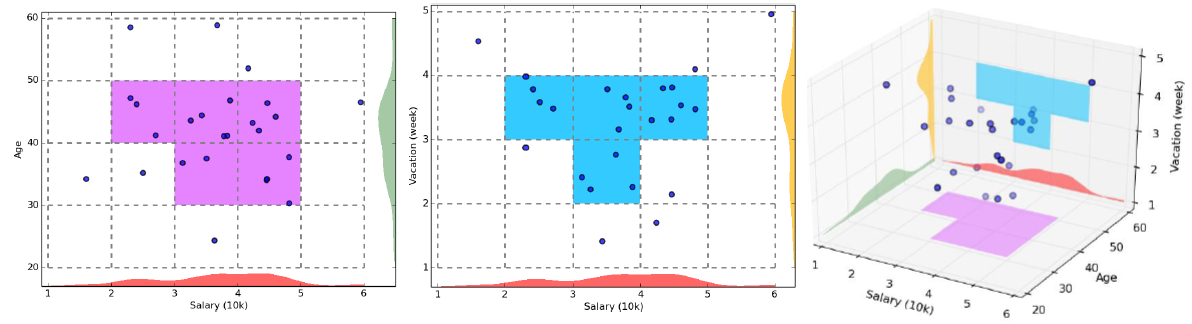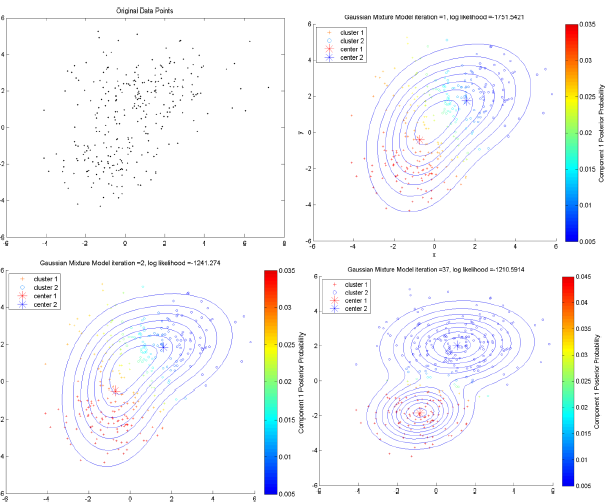# 相似性衡量

## 1. 样本间距离度量

### 1.1 数值变量

$dis_q(\boldsymbol{x},\boldsymbol{y})=[\sum_{k=1}^{m}|x_k-y_k|^q]^{\frac{1}{q}}$

• 曼哈顿(Manhattan)距离：$q=1$

$dis_q(\boldsymbol{x},\boldsymbol{y})=\sum_{k=1}^{m}|x_k-y_k|$

• 欧几里得(Euclid)距离：$q=2$ 时 （坐标轴正交旋后转保持不变

$dis_q(\boldsymbol{x},\boldsymbol{y})=\sqrt{\sum_{k=1}^{m}|x_k-y_k|^2}$

• 切比雪夫(Chebyshev)距离：$q\to+\infty$

$dis(\boldsymbol{x},\boldsymbol{y})=\max_{1\leqslant k\leqslant m}|x_k-y_k|$

• 马氏(Mahalanobis)距离：

$dis(\boldsymbol{x},\boldsymbol{y})=\sqrt{(\boldsymbol{x}-\boldsymbol{y})^{T}\boldsymbol{\Sigma}^{-1}(\boldsymbol{x}-\boldsymbol{y})}$

### 1.2 二元变量

Name 指标1 指标2 指标3 指标4 指标5 指标6
Eirc 1 0 1 1 0 0
Freddie 1 0 0 1 1 1
Dramwig 0 1 1 1 1 0

X\Y 1 0 sum
1 a b a+b
0 c d c+d
sum a+c b+d a+b+c+d

$dis(X,Y)=\frac{b+c}{a+b+c}$

### 1.3 分类变量

• 简单匹配：$dis(X,Y)=\frac{p-m}{p}$ p为类别数，m为配对数
• 二元化：$Categories:\{C_1,C_2,C_3\}$ $\to$ $C_1:\{0,1\}$ ; $C_2:\{0,1\}$ ; $C_3:\{0,1\}$,

### 1.4 有序变量

1. 记序列为$rank\to[1\dots N]$
2. z-scores来标准化 $r_i/N$$r\in [0,1]$
3. 计算马氏(Minkowski)距离

## 2. 类与类间距度量

• 最短距离法（Nearest Neighbor or Single Linkage Method）：

$D(G_1,G_2)=\min_{\tiny{\boldsymbol{x_i}\in G_1,\boldsymbol{y_j}\in G_2}}{dis(\boldsymbol{x_i},\boldsymbol{y_j})}$

• 最长距离法（Farthest Neighbor or Complete Linkage Method）：

$D(G_1,G_2)=\max_{\tiny{\boldsymbol{x_i}\in G_1,\boldsymbol{y_j}\in G_2}}{dis(\boldsymbol{x_i},\boldsymbol{y_j})}$

• 重心法（Centetoid Method）

$D(G_1,G_2)=dis(\boldsymbol{\bar{x}},\boldsymbol{\bar{y}})$

• 类平均法（Group Average Method）(被认为是最常用也最好用的方法其单调性良好，空间扩张/浓缩的程度适中)

$D(G_1,G_2)=\frac{1}{n_1n_2}\sum_{\boldsymbol{x_i}\in G_1}\sum_{\boldsymbol{y_j}\in G_2}dis(\boldsymbol{x_i},\boldsymbol{y_j})$

• 离差平方和法（Sum of Squares Method）又称 Ward 方法

$D_1=\sum_{\boldsymbol{x_i}\in G_1}(\boldsymbol{x_i-\boldsymbol{\bar{x}}})^{T}(\boldsymbol{x_i-\boldsymbol{\bar{x}}}),D_2=\sum_{\boldsymbol{y_j}\in G_2}(\boldsymbol{y_j-\boldsymbol{\bar{y}}})^{T}(\boldsymbol{y_j-\boldsymbol{\bar{y}}})$

$D_{12}=\sum_{\boldsymbol{z_k}\in G_1\cup G_2}(\boldsymbol{z_k-\boldsymbol{\bar{z}}})^{T}(\boldsymbol{z_k-\boldsymbol{\bar{z}}})$

$D(G_1,G_2)=D_{12}-D_1-D_2$

## 3. 指标间距离度量

• 相关系数

$r_{jk}=\frac{\sum\limits_{i=1}^{n}(x_{ij}-\bar{x}_j)(x_{ik}-\bar{x}_k)}{[\sum\limits_{i=1}^{n}(x_{ij}-\bar{x}_j)^2\sum\limits_{i=1}^{n}(x_{ik}-\bar{x}_k)^2]^{\frac{1}{2}}}$

• 夹角余弦

$r_{jk}=\frac{\sum\limits_{i=1}^{n}x_{ij}x_{ik}}{(\sum\limits_{i=1}^{n}x_{ij}^2\sum\limits_{i=1}^{n}x_{ik}^2)^{\frac{1}{2}}}$

# 聚类算法

## K-均值聚类(k-means)

### 步骤

1. 随机得到 K 个初始质心，每一个质心代表一个类
2. 扫描所有样本点，把每个点划归到到离它最近的质心的簇类中
3. 重新计算每个类的质心。
4. 重复 2. 和 3.
5. 直到分类/质心不在发生变化时结束SPSS-分析-分类-K均值聚类

### 缺陷

1. 每次计算需要给定簇数目K
2. 对孤立点（异常点）敏感，（会产生孤立点为单独一类的情况）
3. 对于初始值敏感
4. k-means不能解决非凸（non-convex）数据（不规则形状聚类）

## 层次(系统)聚类(Hierarchical)

### 步骤

1. 迭代步骤
1. 把每个样本作为单独一个类，计算类与类之间的距离，用邻近度矩阵记录
2. 将邻接矩阵中最小的距离连接的类归为一类
3. 根据新的类，更新邻近度矩阵（若以类中样本间最短距离为类距离则不需要更新）
4. 重复2. 3.
5. 到只只剩下一个类的时候，停止
2. 画出聚类谱系图（SPSS自带）
3. 画出聚合系数折线图
1. 对于每个聚合类别，计算每个类 $C_k$ 的畸变程度：$\sum\limits_{\tiny\boldsymbol{x}_i\in C_k}|\boldsymbol{x}_i-\boldsymbol{\bar{x}}|^2$
2. 计算所有类的总畸变程度，即聚合系数：$J=\sum\limits_{k=1}^{K}\sum\limits_{\tiny\boldsymbol{x}_i\in C_k}|\boldsymbol{x}_i-\boldsymbol{\bar{x}}|^2$
3. 以聚合类别数 $K$ 为横坐标，以聚合系数 $J$ 为纵坐标
4. 通过肘部法则（Elbow Method）判断最优聚合数量，得到聚类结果SPSS-分析-分类-系统聚类

## 基于密度的DBSCAN算法

DBSCAN(Density-based spatial clustering of applications
with noise)### 基本概念

• 核心点：在该点半径 $Eps$ 内含的点数不少于 $MinPts$
• 边界点：在该点半径 $Eps$ 内点的数量小于 $MinPts$，但是落在核心点的半径 $Eps$ 邻域内
• 噪音点：既不是核心点也不是边界点的点，即不在核心点的半径 $Eps$ 邻域内的点

DBSCAN密度聚类可视化### 缺点

• 对输入参数 EpsMinpts 敏感，确定参数困难，不同参数相差很大
• 时间复杂度高，处理大量数据时慢

## 根据网格单元的聚类### 步骤

1.将数据空间划分为网格单元
2.依照设置的阈值，判定网格单元是否稠密
3.合并相邻稠密的网格单元为一类

### 特点

• 优点
• 效率快速度快
• 直观易于理解
• 缺点
• 拟合较为粗糙，分类不精确，无法处理不规则分布的数据
• 对输入参数敏感，难以确定方格宽以及稠密阈值，方格宽以及稠密阈值对结果影响很大

## 基于概率的聚类

Model-based methods# 数据简化

• 变换（Data Transformation）
• 离散傅里叶变换（Discrete Fourier Transformation）可以提取数据的频域（frequency domain）信息
• 离散小波变换（Discrete Wavelet Transformation）除了频域之外，还可以提取到时域（temporal domain）信息
• 降维（Dimensionality Reduction）
• 线性方法
• PCA（Principle Component Analysis）
• SVD（Singular Value Decomposition）
• MDS（Multi-Dimensional Scaling）PCA拓展
• 非线性降维的算法
• 流形学习（Manifold Learning）
• ISOMAP、
• LLE（Locally Linear Embedding）、
• MVU（Maximum variance unfolding）、
• Laplacian eigenmaps、Hessian eigenmaps、
• Kernel PCA、
• Probabilistic PCA
• 抽样（Sampling）
• 随机抽样

# 三大步骤的综合

1. 相似性衡量：距离、相似系数、核函数、DTW（dynamic time warping）
2. 聚类算法：Hierarchical methods、Partition-based methods、Density-based methods、Grid-based methods、Model-based methods
3. 数据简化：变换、降维、抽样

# 参考

• 这里非常感谢知乎答主@郭小天的分享给了我很大启发
1. 司守奎 孙兆亮. 数学建模算法与运用[M]. 第2版. 第216页.